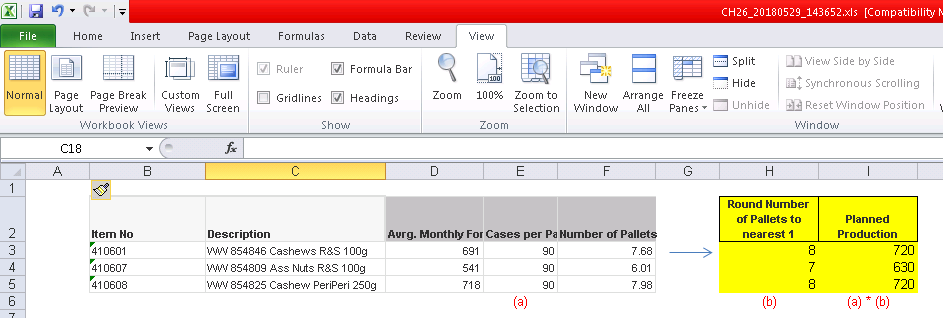# QlikView App Dev

Discussion Board for collaboration related to QlikView App Development.

Announcements
QlikWorld happening right now! You can still join: REGISTER NOW
cancel
Showing results for
Did you mean:Creator

## Rounding up & calculating on object

hi,

i have the following object with calculated fields, i would like to add the calculations marked in yellow.would it be possible to to calculate yellow field?

My column F (number of pallets) calculations is as follows:

(if(IsNull

(sum ([Annual Sales])*-1/12 * (1 + ((sum([3 Months Sales]) - sum([Prior Year 3 Months Sales]))

/

sum([Prior Year 3 Months Sales])))

/

[FG Case weight])

,

(sum([3 Months Sales])*-1/3) / [FG Case weight]

,

(sum ([Annual Sales])*-1/12 * (1 + ((sum([3 Months Sales]) - sum([Prior Year 3 Months Sales]))

/

sum([Prior Year 3 Months Sales])))

/

[FG Case weight]) ) )

/

[FG Pallet Size]

1 Solution

Accepted SolutionsMVP

Should be just using Ceil() function

Ceil(

(if(IsNull

(sum ([Annual Sales])*-1/12 * (1 + ((sum([3 Months Sales]) - sum([Prior Year 3 Months Sales]))

/

sum([Prior Year 3 Months Sales])))

/

[FG Case weight]),

(sum([3 Months Sales])*-1/3) / [FG Case weight],

(sum ([Annual Sales])*-1/12 * (1 + ((sum([3 Months Sales]) - sum([Prior Year 3 Months Sales]))

/

sum([Prior Year 3 Months Sales])))

/

[FG Case weight]) ) )

/

[FG Pallet Size]

)MVP

Should be just using Ceil() function

Ceil(

(if(IsNull

(sum ([Annual Sales])*-1/12 * (1 + ((sum([3 Months Sales]) - sum([Prior Year 3 Months Sales]))

/

sum([Prior Year 3 Months Sales])))

/

[FG Case weight]),

(sum([3 Months Sales])*-1/3) / [FG Case weight],

(sum ([Annual Sales])*-1/12 * (1 + ((sum([3 Months Sales]) - sum([Prior Year 3 Months Sales]))

/

sum([Prior Year 3 Months Sales])))

/

[FG Case weight]) ) )

/

[FG Pallet Size]

)